1、Motivation

（1）参数太多，如果训练数据集有限，很容易产生过拟合；
（2）网络越大、参数越多，计算复杂度越大，难以应用；
（3）网络越深，容易出现梯度弥散问题（梯度越往后穿越容易消失），难以优化模型。

2、Architectural Details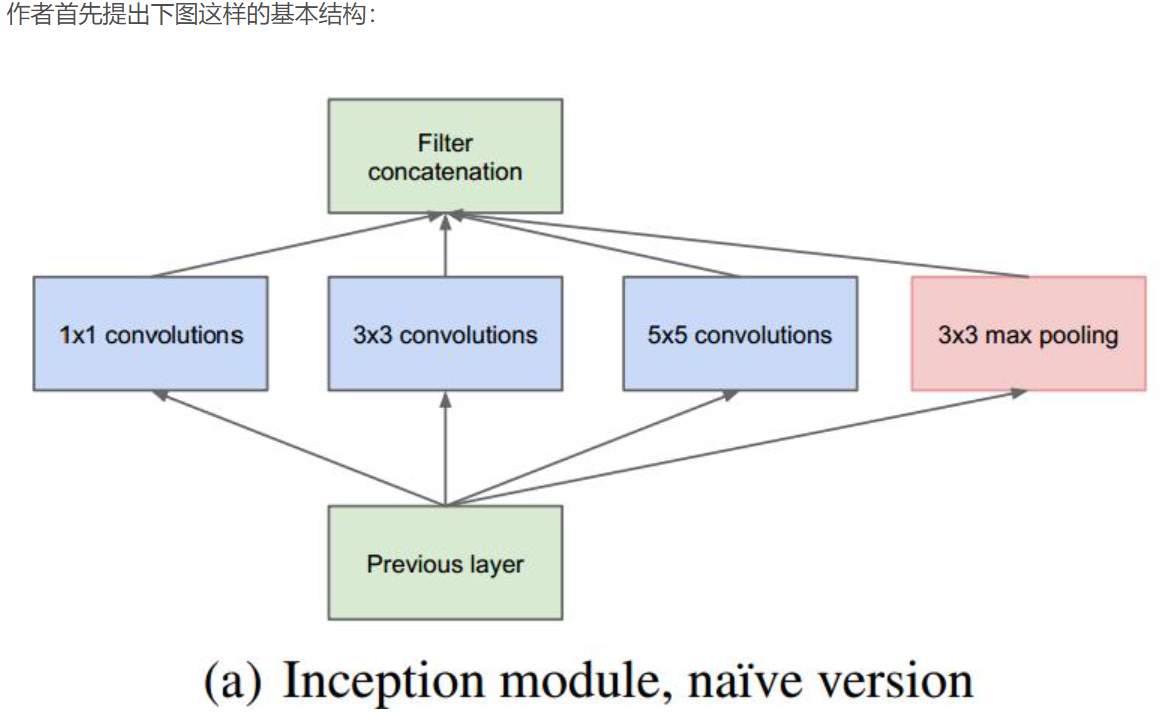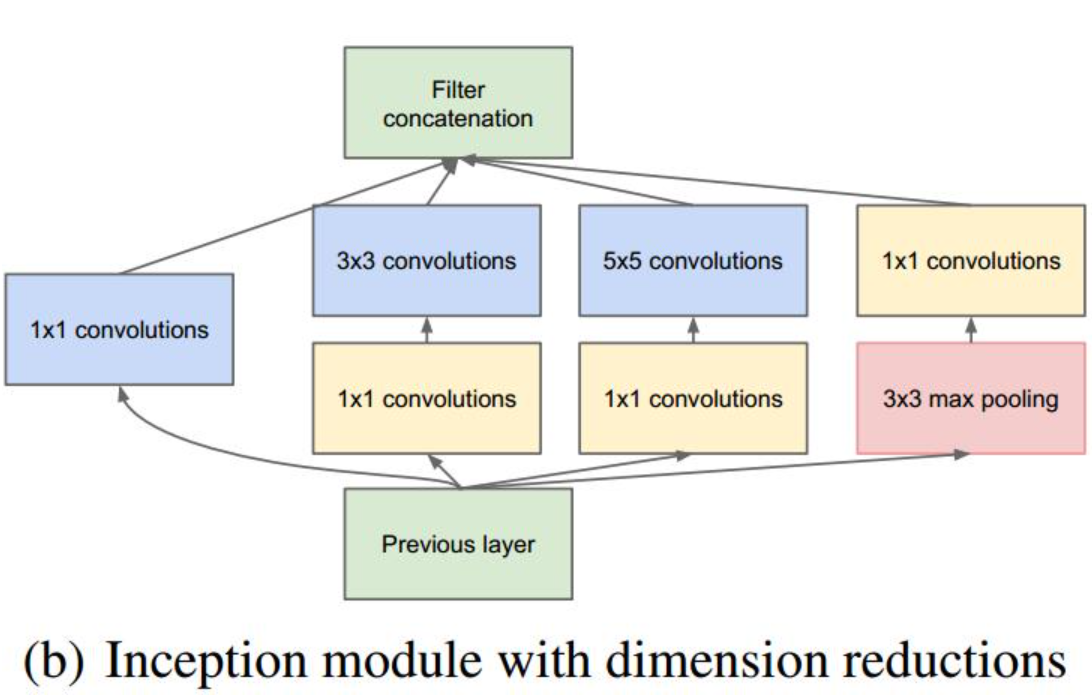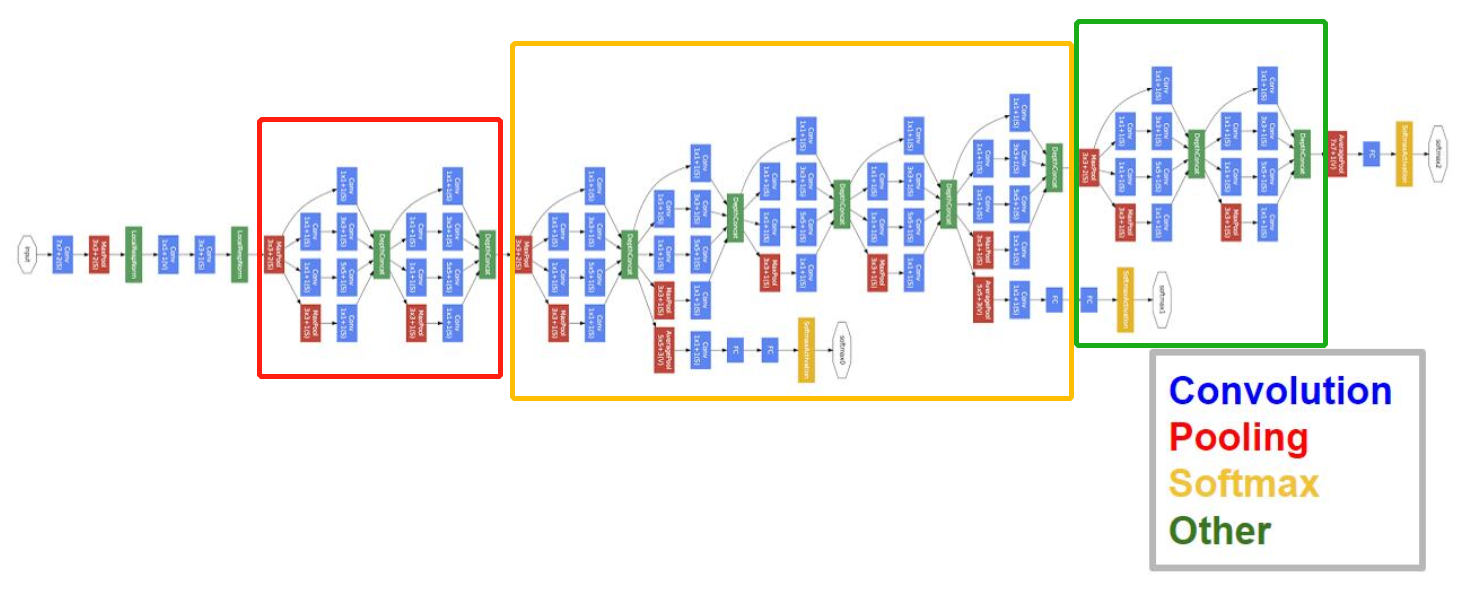2 . 网络最后采用了average pooling来代替全连接层 ,事实证明可以将TOP1 accuracy提高百分之0.6，而且，这样就允许网络接收不同大小的图片输入了，但是，实际在最后还是加了一个全连接层，主要是为了方便以后大家finetune；
3 . 虽然移除了全连接，但是网络中依然使用了Dropout ;
4 . 为了避免梯度消失，网络额外增加了2个softmax辅助分类器，用于向前传导梯度。文章中说这两个辅助的分类器的loss应该加一个衰减系数，但看caffe中的model也没有加任何衰减。此外，实际测试的时候，这两个额外的softmax会被去掉。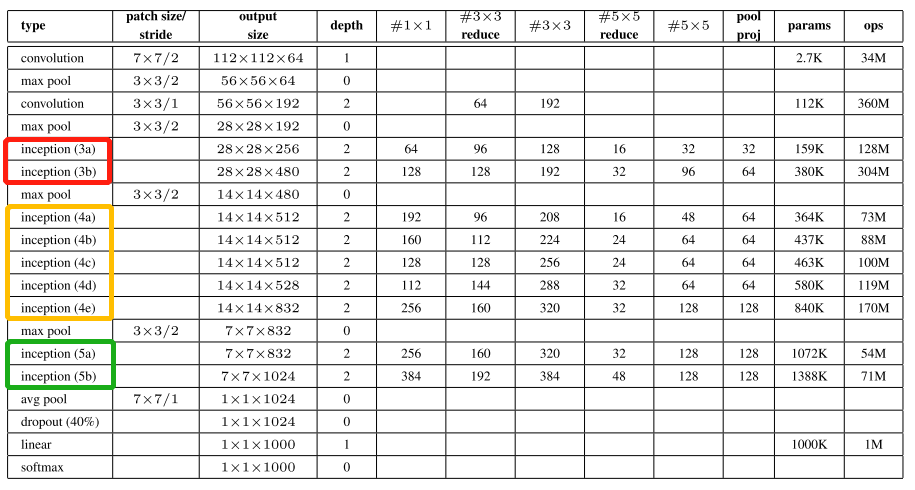1、Motivation

BN使得模型可以使用较大的学习率而不用特别关心诸如梯度爆炸或消失等优化问题；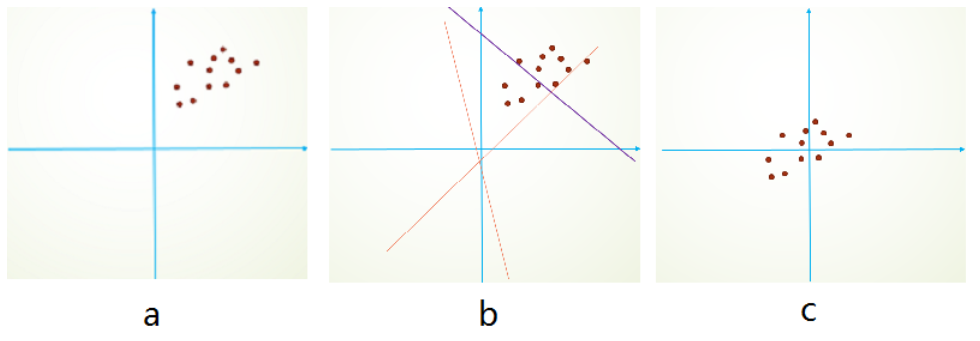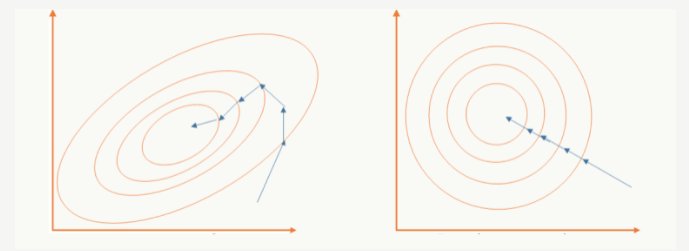2、Architectural Details
V2网络详细参数如下：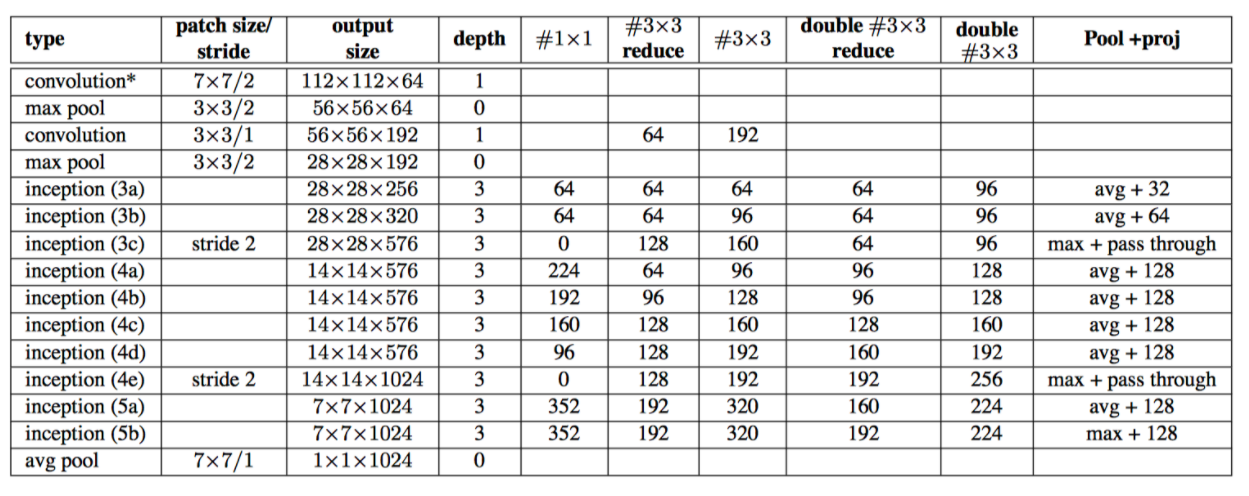GoogLeNet Inception V3在《Rethinking the Inception Architecture for Computer Vision》中提出（注意，在这篇论文中作者把该网络结构叫做v2版，我们以最终的v4版论文的划分为标准），该论文的亮点在于：

1、Motivation

2 、General Design Principles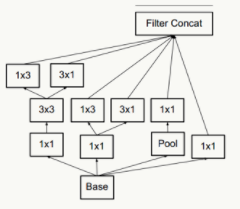inception-v1中提出的用1x1卷积先降维再作特征提取就是利用这点。不同维度的信息有相关性，降维可以理解成一种无损或低损压缩，即使维度降低了，仍然可以利用相关性恢复出原有的信息。

3、Architectural Details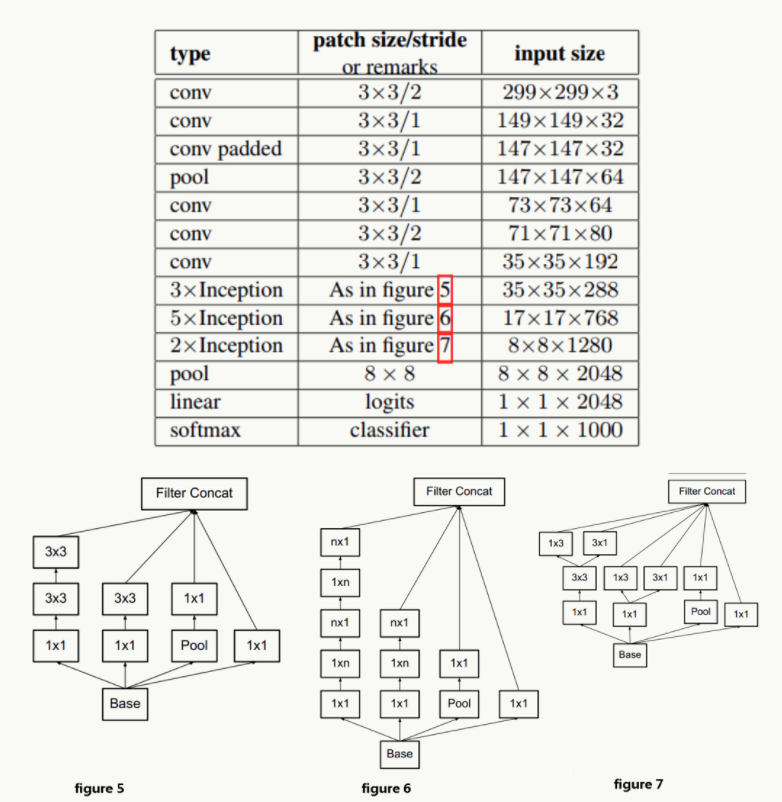figure 5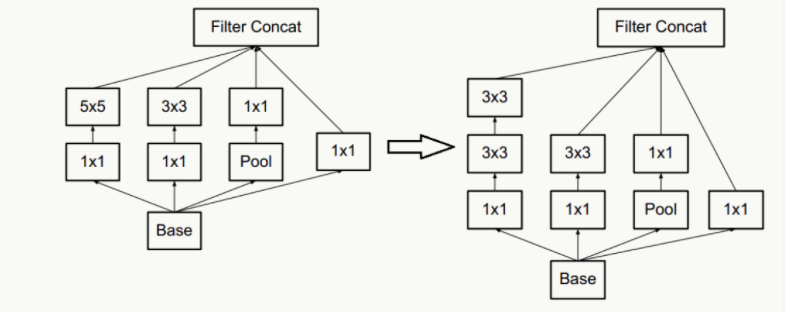figure 6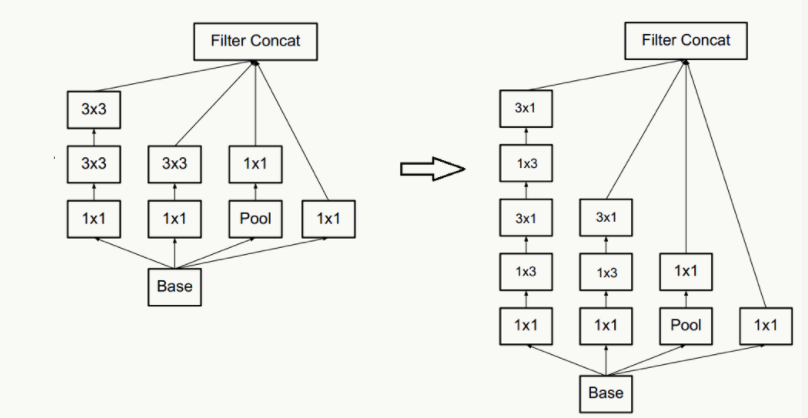figure 7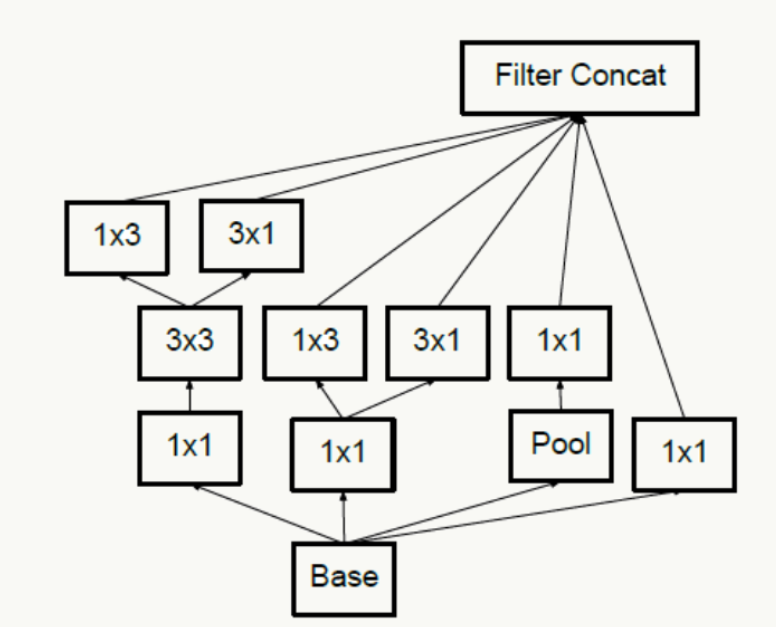V1中的辅助分类器有点问题：auxiliary classifiers在训练初期的时候并不能加速收敛，只有当训练快结束的时候它才会略微提高网络精度。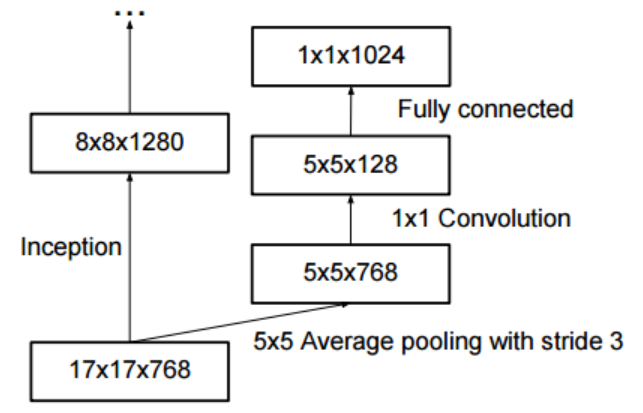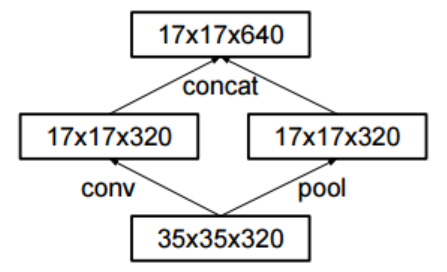Labels的脉冲性质会引发两个不良后果：一是over-fitting，另外一个是降低了网络的适应性。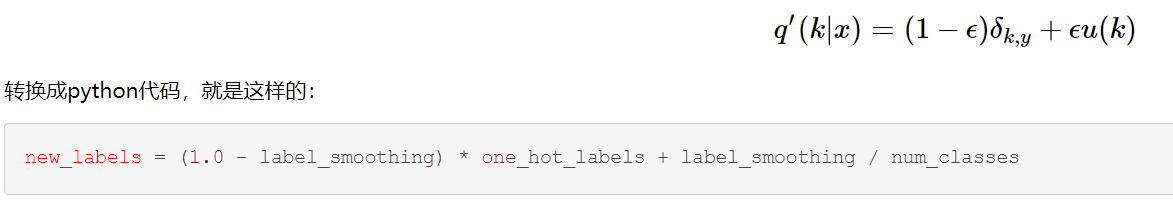Label smooth 把原来很突兀的one_hot_labels稍微的平滑了一点，避免了网络过度学习labels而产生的弊端。

《Inception-v4, Inception-ResNet and the Impact of Residual Connections on Learning》一文中的亮点是：提出了效果更好的GoogLeNet Inception v4网络结构；与残差网络融合，提出效果不逊于v4但训练速度更快的GoogLeNet Inception ResNet结构。from re import S
import torch.nn as nn
import torch
import torch.nn.functional as F

from custom_layers.CustomLayers import ConvActivation, Inception

class Auxiliary_classcification(nn.Module):
def __init__(self, input_channels, num_classes):
super().__init__()
self.avgpool = nn.AvgPool2d(kernel_size=5, stride=3)
self.conv = ConvActivation(input_channels, 128, kernel_size=1) # output[batch, 128, 4, 4]
self.fc1 = nn.Linear(2048, 1024)
self.fc2 = nn.Linear(1024, num_classes)
def forward(self, x):
# aux1: N x 512 x 14 x 14, aux2: N x 528 x 14 x 14
x = self.avgpool(x)
# aux1: N x 512 x 4 x 4, aux2: N x 528 x 4 x 4
x = self.conv(x)
# N x 128 x 4 x 4
x = torch.flatten(x)

x = F.dropout(x, p=0.5, training=self.training)
x = self.fc1(x)
x = F.relu(x, inplace=True)
x = F.dropout(x, p=0.5, training=self.training)
x = self.fc2(x)
return x

def __init__(self, num_classes=None, aux_logits=False, init_weights=False):
super().__init__()
self.aux_logits = aux_logits

self.conv1 = ConvActivation(3, 64, kernel_size=7, stride=2, padding=3)
self.maxpool = nn.MaxPool2d(3, stride=2, ceil_mode=True)

self.conv2 = ConvActivation(64, 192, kernel_size=3, padding=1)
self.maxpool2 = nn.MaxPool2d(kernel_size=3, stride=2, ceil_mode=True)

# input_channels, out_nc1x1, out_nc3x3_reduce, out_nc3x3, out_nc5x5_reduce, out_nc5x5, out_nc_pool
self.inception3a = Inception(192, 64, 96, 128, 16, 32, 32)
self.inception3b = Inception(256, 128, 128, 192, 32, 96, 64)
self.maxpool3 = nn.MaxPool2d(3, stride=2, ceil_mode=True)

self.inception4a = Inception(480, 192, 96, 208, 16, 48, 64)
self.inception4b = Inception(512, 160, 112, 224, 24, 64, 64)
self.inception4c = Inception(512, 128, 128, 256, 24, 64, 64)
self.inception4d = Inception(512, 112, 144, 288, 32, 64, 64)
self.inception4e = Inception(528, 256, 160, 320, 32, 128, 128)
self.maxpool4 = nn.MaxPool2d(3, stride=2, ceil_mode=True)

self.inception5a = Inception(832, 256, 160, 320, 32, 128, 128)
self.inception5b = Inception(832, 384, 192, 384, 48, 128, 128)

if self.aux_logits:
self.aux1 = Auxiliary_classcification(input_channels=512, num_classes=num_classes)
self.aux2 = Auxiliary_classcification(input_channels=528, num_classes=num_classes)

self.dropout = nn.Dropout(0.4)
self.fc = nn.Linear(1024, num_classes)

if init_weights:
self._initial_weights()

def forward(self, x):
x = self.conv1(x)           # [b*3*224*224]  --> [b*64*112*112]
x = self.maxpool(x)         # [b*64*112*112] --> [b*64*56*56]
x = self.conv2(x)           # [b*64*56*56]   --> [b*192*56*56]
x = self.maxpool2(x)        # [b*192*56*56]  --> [b*192*28*28]

x = self.inception3a(x)     # [b*192*28*28]  --> [b*256*28*28]
x = self.inception3b(x)     # [b*256*28*28]  --> [b*480*28*28]
x = self.maxpool3(x)        # [b*256*14*14]  --> [b*480*14*14]

x = self.inception4a(x)     # [b*480*14*14]  --> [b*512*14*14]
if self.training and self.aux_logits:  # eval model lose this layer
aux1 = self.aux1(x)
x = self.inception4b(x)     # [b*512*14*14]  --> [b*512*14*14]
x = self.inception4c(x)     # [b*512*14*14]  --> [b*512*14*14]
x = self.inception4d(x)     # [b*512*14*14]  --> [b*528*14*14]
if self.training and self.aux_logits:   # eval model lose this layer
aux2 = self.aux2(x)
x = self.inception4e(x)     # [b*528*14*14]  --> [b*832*14*14]
x = self.maxpool3(x)        # [b*832*7*7]    --> [b*832*7*7]

x = self.inception5a(x)     # [b*832*7*7]    --> [b*832*7*7]
x = self.inception5b(x)     # [b*832*7*7]    --> [b*1024*7*7]

x = self.avgpool(x)         # [b*1027*7*7]   --> [b*1024*1*1]
x = torch.flatten(x, 1)     # [b*1027*1*1]   --> [b*1024]
x = self.fc(x)              # [b*1024]       --> [b*num_classes]

if self.training and self.aux_logits:   # eval model lose this layer
return x, aux2, aux1
return x

def _initial_weights(self):
for m in self.modules():
if isinstance(m, nn.Conv2d):
nn.init.kaiming_normal_(m.weight, mode='fan_out', nonlinearity='relu')
if m.bias is not None:
nn.init.constant_(m.bias, 0)
elif isinstance(m, nn.Linear):
nn.init.normal_(m.weight, 0, 0.01)
nn.init.constant_(m.bias, 0)